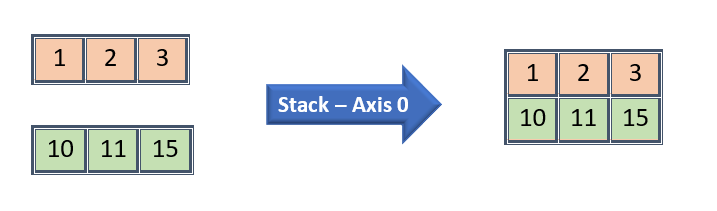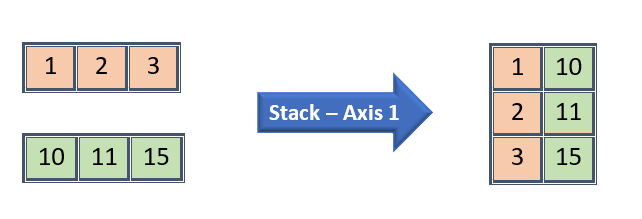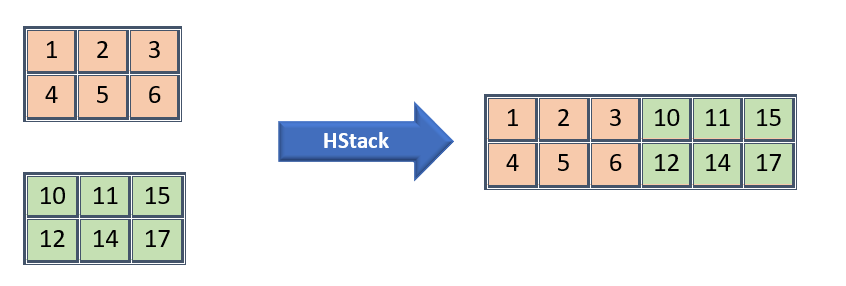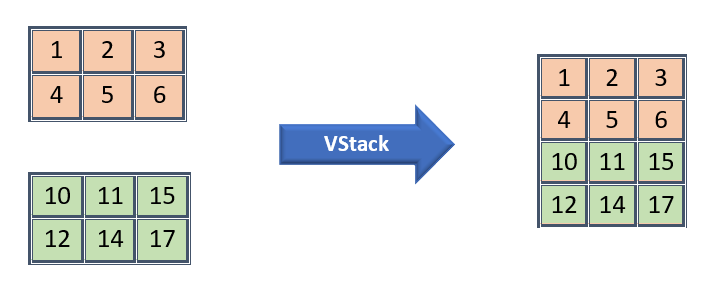# Numpy — Stacking Arrays

### Joining two numpy arrays

• stack — Joins arrays with given axis element by element
• hstack — Extends horizontally
• vstack — Extends vertically

### Stack — Joins arrays with given axis element by element

• Both input arrays should be in same dimension/shape
• Axis parameter in Stack works as dimension here instead of horizontal/vertical manner.
• If Axis is 0, then it will join by first dimension
• If Axis is 1, the it will join by second dimension
• The maximum dimension that we can mention is dimension of input arrays (say n) + 1.
• If axis is given above n + 1, then “out of bounds for array of dimension” exception will be thrownStack by First DimensionStack by Second dimenstion

Output:

Stack by First Dimension:

Output:

Stack by Second Dimension:

Output:

### HStack — Stacks horizontally

• This function does not work with axis. It extends first array by second array Horizontally
• As it extends horizontally, both the arrays should have same number of rows else Value Error will be returned.HStack

HStack for 1D Arrays:

Output:

HStack for 2D Arrays:

Output:

### VStack — Stacks vertically

• This function does not work with axis. It extends first array by second array VerticallyVStack

VStack by 2D Arrays:

Output:

### Conclusion:

In this article we experimented 1D and 2D arrays for stacking.

I will be adding another post with more demonstration for 3D arrays as it is little tricky to understand.

If you like this article and support me, just click the heart icon!!!

Subscribe to our site to get notified for new post.

Happy Programming!!!

Like to support? Just click the heart icon ❤️.

0